Courses

# Test : Geometric Shapes - 2

## 10 Questions MCQ Test Mathematics for Class 5 (V) - CBSE and NCERT Curriculum | Test : Geometric Shapes - 2

Description
This mock test of Test : Geometric Shapes - 2 for Class 5 helps you for every Class 5 entrance exam. This contains 10 Multiple Choice Questions for Class 5 Test : Geometric Shapes - 2 (mcq) to study with solutions a complete question bank. The solved questions answers in this Test : Geometric Shapes - 2 quiz give you a good mix of easy questions and tough questions. Class 5 students definitely take this Test : Geometric Shapes - 2 exercise for a better result in the exam. You can find other Test : Geometric Shapes - 2 extra questions, long questions & short questions for Class 5 on EduRev as well by searching above.
QUESTION: 1

###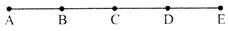denotes How Many line segments.

Solution: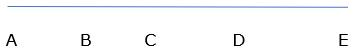10 line segments are there, namely
AB, AC, AD, AE, BC, BD, BE, CD, CE, DE

QUESTION: 2

### If 2/3 AB = 7/2 BC = 42cm then

Solution:

AB = 3 x 42/2 = 63 cm
BC = 2 x 42/7 = 12 cm
Therefore, AB>BC

QUESTION: 3

### Line segment AB is congruent to line segment CD, it is represented as

Solution:

Congruency means that line segments AB and CD are equal in all respects.

QUESTION: 4

The unit of measure of an angle is

Solution:

Unit of measure of an angle is degrees.

QUESTION: 5

The sum of all angle of triangle is ___________

Solution:

Sum of all angles of a triangle is 180°.

QUESTION: 6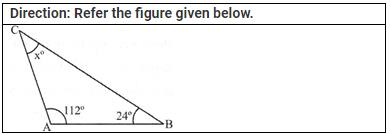Find xo=?

Solution:

Sum of all angles of a triangle is 180°.
Therefore, x = 180 – (112+24) = 44°

QUESTION: 7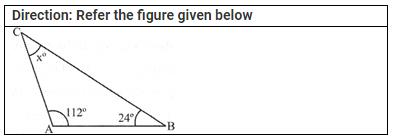∠A+∠C=?

Solution:

Angle A + Angle B + Angle C = 180°
Therefore, Angle A + Angle C = 180 -24 = 156°

QUESTION: 8∠C−∠B=?

Solution:

Sum of all angles of a triangle is 180°.
Therefore, Angle C = x = 180 – (112+24) = 44°
Angle C – Angle B = 44 – 24 = 20°

QUESTION: 9​2∠A−(∠B+∠C)=?

Solution:

Sum of all angles of a triangle is 180°.
Therefore, Angle C = x = 180 – (112+24) = 44°
2(Angle A) – (Angle B + Angle C) = 2x112 – (24+44)
= 224 – 68
= 156°

QUESTION: 10

In a ΔABC, if ∠B=90o , then ________

Solution: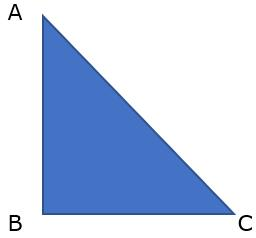If Angle B = 90°, then Triangle ABC is a Right Angled Triangle.
Therefore,  AC2 = AB2 + BC2